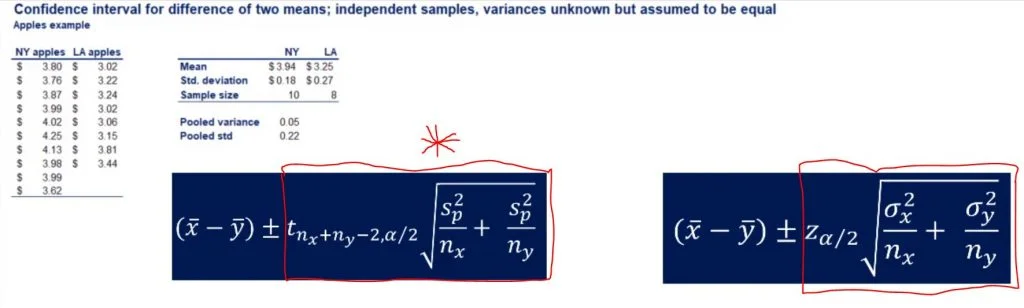25 Jan 2021

Posted on:

08 Dec 2020

0

# Review Project Al Bundy show shopPlease explain to me why you are square rooting the pooled variance, divided by the sample size plus the other sample size, and then dividing again by the confidence interval when you clearly did not teach that. That is not how it shows to calculate the confidence interval of two independent variables with variances unknown and assumed to be equal. NO WHERE does it say to use the margin of error to calculate it and no where does it say to sqrt the pooled variance, divided by the sample size plus the other sample size, and then dividing again by the confidence interval.
This is not the first time I have come across a situation where your lecture has said one thing and then in examples you clearly did something against what you have said. This is beyond frustrating when trying to learn something. If you are going to change the equation completely when calculating it then YOU NEED TO STATE that in the lecture. That's poor teaching!

The margin of error is not always calculated the same way. It depends on whether we're using the Z-Statistic or the T-Statistic, whether we know the population variance, whether we have one or more samples and whether those samples are dependent or independent. However the margin of error does always (as far as I know) consist of the product of a certain statistic and an expression with variance(s) and sample size(s).   Having said all of this, saying they "divided by the confidence interval" is a mistake, as they cannot divide by something they're trying to calculate. On the other hand, they did exactly what's in the first picture you provided and in the "formulas for confidence intervals" part of the section 2 course notes.    in this particular case,The expressions in the red squares are both margins of error, with the one with the big ugly red asterisk being the one used for this practical example.   I hope this was enough to clear things up. Please let me know it either I misunderstood or if you want me to elaborate further.    Thanks.